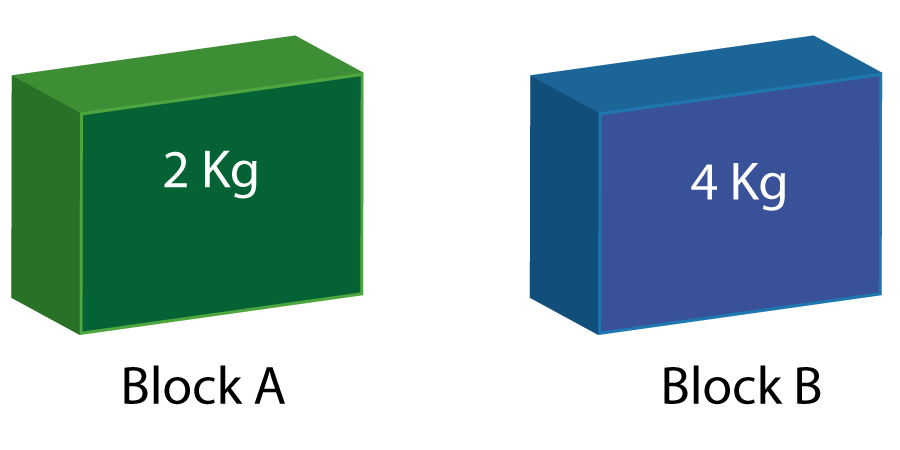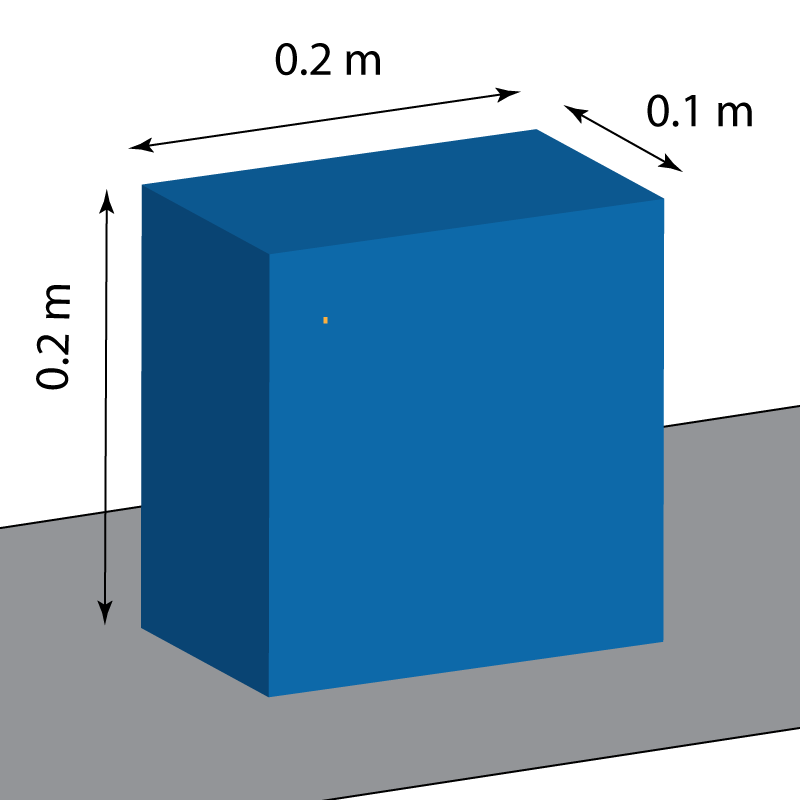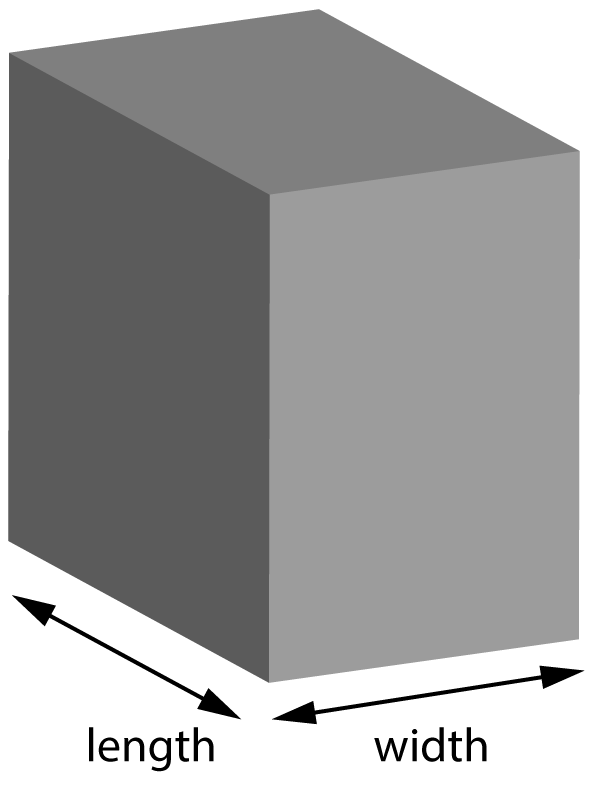NEED HELP? Edexcel iGCSE digital textbooks From the makers of Grade Gorilla. GGebooks.com1. Which answer best describes the difference between these 2 blocks?• A) Block A has a larger volume than block B
• B) Block B is denser than block A
• C) Block A has more mass than block B
• D) Block A has more tightly packed particles than block B

2. What is the formula for density?

• A) Density = volume x mass
• B) Density = volume ÷ mass
• C) Density = mass ÷ volume
• D) Density =  ½ x mass x (volume)

3. A block of steel has a volume of 50 cm3, a length of 4cm and a width of 5cm. The height of the block is

• A) 2 cm
• B) 2.5 cm
• C) 5 cm
• D) 10 cm

4-6: What are the missing values in this table?

 Substance Mass (g) Volume (cm3) Density (g/cm3) 4. Wood 20 25 ? 500 50 0.8 1.25 5. Copper ? 324 4 0.25 25 36 9 6. Glass 400 ? 100 160 800 1000 2.5

7.. Which of these is the correct formula to calculate the pressure on an area?

• A) P = F x A
• B) P = F ÷ A
• C) P = F x d
• D) P = F ÷ d

8+9: A block of mass 3000 g rests on a desk as shown.8. What is the weight of the block?

• A) 0.3 kg
• B) 3 kg
• C) 3 N
• D) 30 N

9. What pressure does the block cause on the desk?

• A) 1500 Pa
• B) 150 Pa
• C) 7500 Pa
• D) 750 Pa

10-12:

What are the missing values in this table showing the pressure under different steel blocks in a scrap yard?Length (m) Width (m) Area (m2) Force on ground (N) Pressure (Pa) 0.8 0.5 0.4 20 000 ? 8 000 50 000 500 10 000 ? 2 20 0.1 10 0.2 2 ? 2500 10 000 25 000 100 000 5000